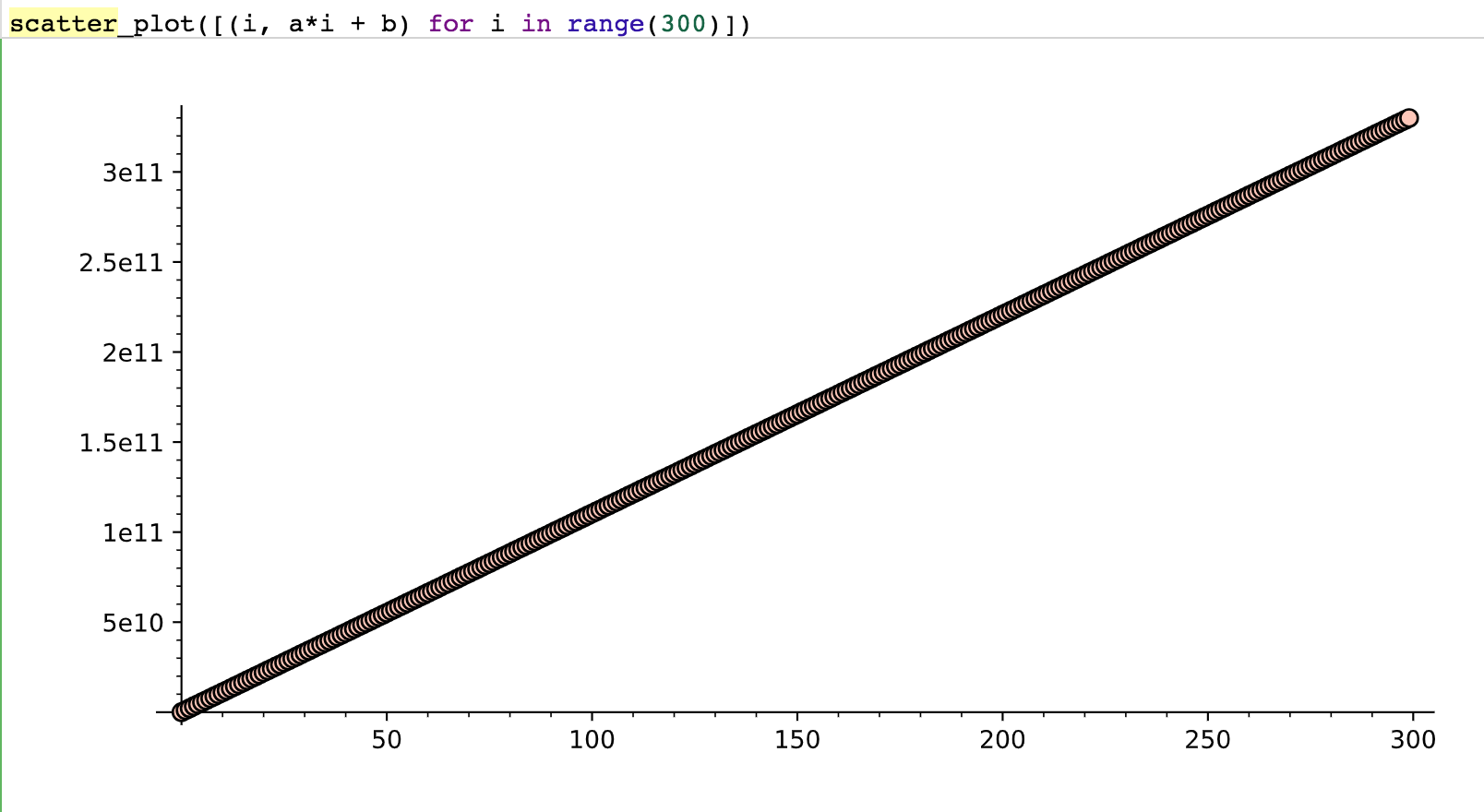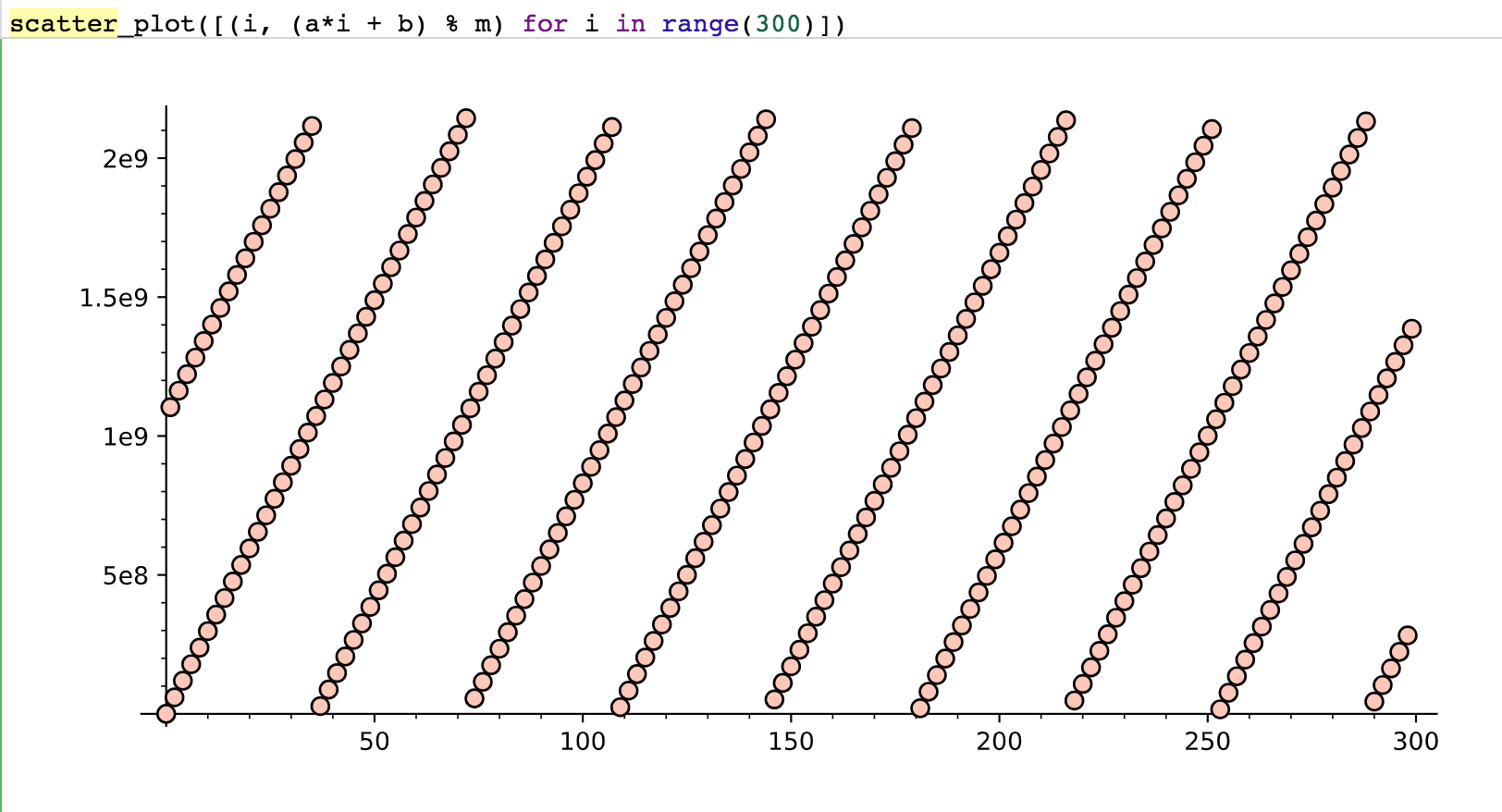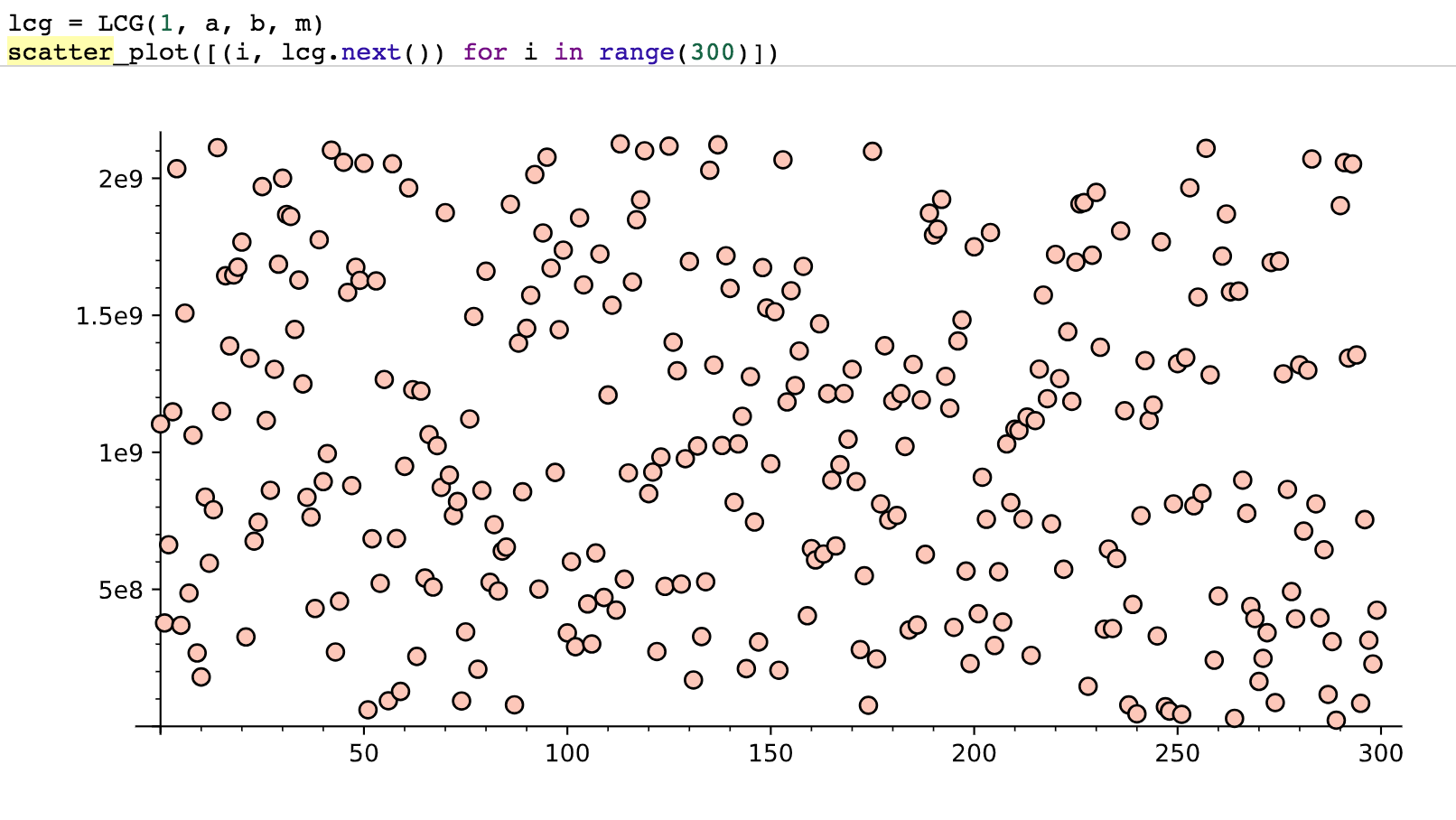# Class 6: Pseudo-randomness

Overview: This lesson is centered around pseudo-random number generation (actually this lesson centers around insecure pseudo-random number generators). The goal of a PRNG is to have a reproducible sequence of random feeling numbers. There are a lot of reasons to do this including running simulations of markets and experiments, rolling dice in games, and our application will be for generating symmetric key encryption. We will start with some classic PRNGs and start to break them over time. In this part I'll show you how to think about the security of a PRNG.

# Pseudo-random Generators / Functions / Permutations

As we start to explore private-key crypto it's important to see how the pieces fit together. How do we know when a scheme is leaking information? How do we analyze the schemes?

We will be playing with stream ciphers which is essentially the same as playing with pseudo-random number generators. Here are two properties that help us define a CSPRNG (Cryptographically Secure Pseudo Random Number Generator):

• Next-bit test: Given $$n$$ bits of output you should be able to guess the next-bit with no better than 50% probability. Given $$s_{i+1}, s_{i+2}, \ldots, s_{i+n}$$ you shouldn't be able to guess $$s_{i+n+1}$$
• State Compromise Extension: Given the bits $$s_{i+1}, \ldots, s_{i+n}$$ it should be computationally infeasible to calculate $$s_{i}, s_{i-1}, \ldots$$.

#### Which rule violation?:

The following 10 pseudo-random chunks were made by me using some structured rules. Can you distinguish them from true random? Which of the above two rules are violated? (Answers hidden in the HTML.) There is an even number of 1s, the last digit was generated as a "parity check". So if you knew the first 7 bits you would also know the last bit.

• 10011001
• 01010110
• 10000100
• 01011100
• 11101011
• 00011101
• 10110001
• 11101110
• 10001110
• 00010010

### The first Pseudo-Random generator

In the early days of computing we needed to simulate nuclear reactions, but if there was a bug in the code we had to be able to rerun the randomized simulations. So von Neumann used the following simple method: the "middle-square" method.

To generate a sequence of n-digit numbers pick one out as your "seed". Square it. Take the middle n-digits as the next number. For instance, if your seed is 682117, then square it and get 465283601689, so the next term is 283601. Square and repeat.

Try it out: Try to implement the middle squares method for 6-digit numbers in Python. If the square is too short add zeros to the top.

Predictability: So which of the above rules does this violate? (It's terrible for cryptography, but why?)

### Web Scraping Aside:

This is just a fun thing, won't be tested and it will be tough. BUT you'll be very happy you worked through it.

This is just a fun super-power to add to your abilities. The following snippet scrapes this site with digits of pi in binary and gets the 8-bit chunks into an array.

If you are feeling like using cooler tools try  sudo pip install BeautifulSoup4  to use BeautifulSoup.

If you've never played with regular expressions before then take a look at some docs .

Practice Scraping Data: Create an array of the Chuck Norris jokes on this page: ICNDb.com .

### Von Neumann Extractor

OK, so if you get a somewhat random chunk of bits from reality and you want to smooth them in a uniformly random way then do the following:

This will return something much closer to an even number of 0s and 1s but it will still be random if your input bits were random.

Uniformize Pi: Now take the chunks you scraped earlier from binary pi and extract a more uniformly random sequence of bits.

#### Analyze the random:

Your sequence of uniformized pi bits come from an interesting real number. Which of the above two CSPRNG properties does it violate?

Overview We now begin looking at existing PRNGs that are really used and that are NOT good enough for cryptographic purposes. We will break some of these schemes using math in the next lesson. Breaking the actual rand function from gcc will be your big assignment for this module. For the rest of this lesson we'll show you modern PRNGs that are great just not for crypto.

So the following is our first somewhat common PRNG that is not cryptographically secure.

Linear congruential generators work off of the recurrence:

$$R_{i+1} \equiv a \cdot R_{i} + c \mod{m}$$

The seed is $$R_0$$ and the key is $$(a,c)$$, $$m$$ is part of the scheme and is known (normally $$m$$ is a 100-bit number that makes computation mod $$m$$ as fast as possible, like a power of 2).

Some old  gcc  (common C compilers) uses $$m = 2^{31}, a=1103515245, c=12345$$ (and they output the bits 30 down to 0).Try it out: Use the seed 3 and the values above and compute the first 5 terms.

Break an LCG: Using the above $$m,a,c$$ I chose an R0 seed (I'm giving all bits of the output, btw). The first 4 outputs were: R1 = 300562173, R2 = 1109962738, R3 = 404411971, R4 = 730322112. Find my seed (and thus the rest of my random number stream). (Try your best at this point, if you don't win come back after lesson 3.)

#### NEW CTF FLAG:

Overview: In this part I introduce the mechanics of the world's most used PRNG, the glibc implementation of rand(). I will show you in the next lesson how to break it under certain situations and your job will be to break it a more general case (observe a sequence of outputs then predict all of the rest of the outputs).

So the following script is how the majority of pseudo-random numbers in the world are generated. From the open-source gcc C-compiler. I have no research to back that up, but I think there is more C code in the world than anything else. This is the basic way (we don't have to know C but you could run this on a normal terminal using gcc crand.c then ./a.out).

Now the following Python script will put out the exact same 5 numbers. This shows the code under the hood and if you see them both you'll feel very confident that we have the right idea.

There is one weird bit here which is that when we print the numbers we have to adjust for the fact that C uses 32-bit numbers and has to also keep negatives with only 32 bits. So that bizarre last line (and the  % 2**32  parts) are just to mimic C.

Confirm: Try running both of these programs using several different seeds. When both programs use the same seed ensure that you get the same values.

The basic pattern is like the linear congruential generator but using older terms and not just the most recent term. We're going to break this sequence in this module (get a sequence of numbers from this generator then figure out the seed).

Overview: this is another classic PRNG which is used in many situations around the globe. It's not good enough for us and we have to break it to see why.

## Linear Feedback Shift Registers

So this is a simple way to make a stream of bits which behaves well for a while.The idea is simple enough, there is a "state" of the register which is the current bits held in each position. There is an "output" bit which will be the right-most bit in this diagram. There is an "input" bit which is an XOR of some of the current bits.

Let's walk through the first couple of outputs when the initial state is 0110:

i State Next Output
1 0110 0
2 1011 1
3 0101 1

Here the output bit it the right-most and the new input bit is the XOR of the two right-most bits, the other 3 bits shift to the right. Each state uniquely determines the next state, so when you repeat a state you've found a "cycle".

Continue: Keep generating one bit at a time, shifting, and XORing until you get to a state which has been seen before. How many distinct states did it take on?

Worst Case: Can you think of a fixed state? That is, a state which will automatically generate itself as the next state?

### Generalized LFSR

In our first example we have two bits which provide "feedback" by XORing to create the new input bit. We could certainly have more bits that feedback to create the new input. In fact each bit can choose if it will contribute or not.

So to make a fully generic LFSR it would look like this:In this diagram each $$c_i$$ is a "coefficient" which will be 0 or 1.

This diagram also has $$L$$ state bits. So we could write a linear recurrence for this which looks like:

$$s_{L} = c_1 \cdot s_{L-1} \oplus \cdots \oplus c_L \cdot s_{0}$$

That can be generalized to:

$$s_{L+j} = c_1 \cdot s_{L-1+j} \oplus \cdots \oplus c_L \cdot s_{j}$$

Key Space: So for a length $$L$$ linear feedback shift register, how many bits are needed to know the entire stream?

Cycle Length: What is the longest cycle of bits for a length $$L$$ LFSR?

Funky Cycle: Now imagine the LFSR of length 4 with feedback coefficients 0,1,0,1 ($$s_{4+j} = s_{2+j} + s_{j}$$) and starting state 0110. How long is the cycle?

Sometimes the setup of the LFSR is conveyed using a polynomial, the one from the previous example would be given as $$x^4 + x^2 + 1$$.

Break an LFSR: I built a length 5 LFSR and the first 10 output bits were 1100100110. Tell me the next 10 bits. (HINT: see these as a system of linear equations.) If you struggle with this just come back after the next lesson.